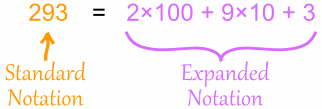Definition of

# Expanded NotationWriting a number to show the value of each digit.

It is shown as a sum of each digit multiplied by its matching place value (ones, tens, hundreds, etc.)

For example: 4,265 = 4 × 1,000 + 2 × 100 + 6 × 10 + 5 × 1